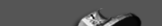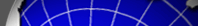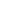Home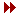ScienceInstrumentationSouthPole SiteObserving & AnalysisSPT TeamNews & PublicationsInternal pagesProject Supported by NSF, National Science Foundation KICP, Kavli Institute for Cosmological Physics USAP, United States Antarctic Program Raytheon Polar Support Corporation

 Related Websites CBI, Cosmic Background Imager DASI, Degree Angular Scale Interferometer WMAP, Wilkinson Microwave Anisotropy ProbeOverviewPoint source catalogDifferential Source Counts at 95 GHzDifferential Source Counts at 150 GHzDifferential Source Counts at 220 GHzCumulative Source Counts at 95 GHzCumulative Source Counts at 150 GHzCumulative Source Counts at 220 GHzCovariance matrices for number countsOverviewThis page provides data products associated with the point-source catalog from 2530 square degrees of SPT data described in Everett et al. (2020). Linked below are the full three-band source catalog (down to a significance threshold of 4.5 sigma), the differential number counts in each band---total and divided into synchrotron- and dust-dominated counts---and the cumulative number counts in each band.CatalogThe full 4.5-sigma source catalog at 95, 150, and 220 GHz from Everett et al. (2020) is here .

Column descriptions from Everett et al. 2020:

1. Source ID: the IAU designation for the SPT-detected source.
2. RA: right ascension (J2000) in degrees.
3. DEC: declination (J2000) in degrees.
4. S/N (95 GHz): detection significance (signal-to-noise ratio) in the 95 GHz band.
5. Sraw (95 GHz): raw flux (uncorrected for flux boosting) in the 95 GHz band.
6. Sbest (95 GHz): median value of de-boosted flux at 95 GHz.
7. dSup (95 GHz): upper error bar (1-sigma normal equivalent) of de-boosted flux at 95 GHz.
8. dSdown (95 GHz): lower error bar (1-sigma normal equivalent) of de-boosted flux at 95 GHz.
9. S/N (150 GHz): detection significance (signal-to-noise ratio) in the 150 GHz band.
10. Sraw (150 GHz): raw flux (uncorrected for flux boosting) in the 150 GHz band.
11. Sbest (150 GHz): median value of de-boosted flux at 150 GHz.
12. dSup (150 GHz): upper error bar (1-sigma normal equivalent) of de-boosted flux at 150 GHz.
13. dSdown (150 GHz): lower error bar (1-sigma normal equivalent) of de-boosted flux at 150 GHz.
14. S/N (220 GHz): detection significance (signal-to-noise ratio) in the 150 GHz band.
15. Sraw (220 GHz): raw flux (uncorrected for flux boosting) in the 220 GHz band.
16. Sbest (220 GHz): median value of de-boosted flux at 220 GHz.
17. dSup (220 GHz): upper error bar (1-sigma normal equivalent) of de-boosted flux at 220 GHz.
18. dSdown (220 GHz): lower error bar (1-sigma normal equivalent) of de-boosted flux at 220 GHz.
19. alpha95-150raw: estimate (from the raw flux in each band) of the 95 GHz-150 GHz spectral index alpha
20. alpha95-150best: median value of spectral index.
21. d_alpha95-150up: upper error bar (1-sigma normal equivalent) of spectral index.
22. d_alpha95-150down: lower error bar (1-sigma normal equivalent) of spectral index.
23. alpha150-220raw: estimate (from the raw flux in each band) of the 150 GHz-220 GHz spectral index alpha
24. alpha150-220best: median value of spectral index.
25. d_alpha150-220up: upper error bar (1-sigma normal equivalent) of spectral index.
26. d_alpha150-220down: lower error bar (1-sigma normal equivalent) of spectral index.
27. P(alpha > 1.51): fraction of the spectral index posterior probability distribution above the threshold value of 1.51. A higher value of P means the source is more likely to be dust-dominated.
28. Type: source classification ((sync)hrotron- or (dust)-dominated).
29. External counterparts: (1): SUMSS, (2): IRAS, (3): AT20G, (4): WISE, (5): PMN, (6): AKARI/FIS, (7): AKARI/IRC, (8): RASS
30. Extended flag: flag for extended sources.
31. Redshift: cross-matched redshift from NED, if available.
32. Cut selection: (1): Ext cut, (2): z cut, (3): SMG list, (4): starsDifferential Source Counts at 95 GHzThe differential number counts as a function of 95 GHz flux from Everett et al. (2020) are here .

Columns in the file are:

1. Flux range [low]: Low end of flux bin in which counts were estimated. In units of Jy.
2. Flux range [high]: High end of flux bin in which counts were estimated. In units of Jy.
3. dN/dS total no cuts, central: Central value of differential counts (in units of number per square degree per Jy) for all sources detected at 95 GHz.
4. dN/dS total no cuts, lower error bar: 1-sigma lower error bar for differential counts (in units of number per square degree per Jy) for all sources detected at 95 GHz.
5. dN/dS total no cuts, upper error bar: 1-sigma upper error bar for differential counts (in units of number per square degree per Jy) for all sources detected at 95 GHz.
6. dN/dS synchrotron z cut, central: Same as  for synchrotron-dominated sources detected at 95 GHz.
7. dN/dS synchrotron z cut, lower error bar: Same as  for synchrotron-dominated sources detected at 95 GHz.
8. dN/dS synchrotron z cut, upper error bar: Same as  for synchrotron-dominated sources detected at 95 GHz.
9. dN/dS dust z cut, central: Same as  for dust-dominated sources detected at 95 GHz.
10. dN/dS dust z cut, lower error bar: Same as  for dust-dominated sources detected at 95 GHz.
11. dN/dS dust z cut, upper error bar: Same as  for dust-dominated sources detected at 95 GHz.
12. Completeness: Average value of completeness across the flux range given by  and .Differential Source Counts at 150 GHzThe differential number counts as a function of 150 GHz flux from Everett et al. (2020) are here .

Columns in the file are:

1. Flux range [low]: Low end of flux bin in which counts were estimated. In units of Jy.
2. Flux range [high]: High end of flux bin in which counts were estimated. In units of Jy.
3. dN/dS total no cuts, central: Central value of differential counts (in units of number per square degree per Jy) for all sources detected at 150 GHz.
4. dN/dS total no cuts, lower error bar: 1-sigma lower error bar for differential counts (in units of number per square degree per Jy) for all sources detected at 150 GHz.
5. dN/dS total no cuts, upper error bar: 1-sigma upper error bar for differential counts (in units of number per square degree per Jy) for all sources detected at 150 GHz.
6. dN/dS synchrotron z cut, central: Same as  for synchrotron-dominated sources detected at 150 GHz.
7. dN/dS synchrotron z cut, lower error bar: Same as  for synchrotron-dominated sources detected at 150 GHz.
8. dN/dS synchrotron z cut, upper error bar: Same as  for synchrotron-dominated sources detected at 150 GHz.
9. dN/dS dust z cut, central: Same as  for dust-dominated sources detected at 150 GHz.
10. dN/dS dust z cut, lower error bar: Same as  for dust-dominated sources detected at 150 GHz.
11. dN/dS dust z cut, upper error bar: Same as  for dust-dominated sources detected at 150 GHz.
12. dN/dS SMG, central: Same as  for SMG list sources detected at 150 GHz.
13. dN/dS SMG, lower error bar: Same as  for SMG list sources detected at 150 GHz.
14. dN/dS SMG, upper error bar: Same as  for SMG list sources detected at 150 GHz.
15. Completeness: Average value of completeness across the flux range given by  and .Differential Source Counts at 220 GHzThe differential number counts as a function of 220 GHz flux from Everett et al. (2020) are here .

Columns in the file are:

1. Flux range [low]: Low end of flux bin in which counts were estimated. In units of Jy.
2. Flux range [high]: High end of flux bin in which counts were estimated. In units of Jy.
3. dN/dS total no cuts, central: Central value of differential counts (in units of number per square degree per Jy) for all sources detected at 220 GHz.
4. dN/dS total no cuts, lower error bar: 1-sigma lower error bar for differential counts (in units of number per square degree per Jy) for all sources detected at 220 GHz.
5. dN/dS total no cuts, upper error bar: 1-sigma upper error bar for differential counts (in units of number per square degree per Jy) for all sources detected at 220 GHz.
6. dN/dS synchrotron z cut, central: Same as  for synchrotron-dominated sources detected at 220 GHz.
7. dN/dS synchrotron z cut, lower error bar: Same as  for synchrotron-dominated sources detected at 220 GHz.
8. dN/dS synchrotron z cut, upper error bar: Same as  for synchrotron-dominated sources detected at 220 GHz.
9. dN/dS dust z cut, central: Same as  for dust-dominated sources detected at 220 GHz.
10. dN/dS dust z cut, lower error bar: Same as  for dust-dominated sources detected at 220 GHz.
11. dN/dS dust z cut, upper error bar: Same as  for dust-dominated sources detected at 220 GHz.
12. dN/dS SMG, central: Same as  for SMG list sources detected at 220 GHz.
13. dN/dS SMG, lower error bar: Same as  for SMG list sources detected at 220 GHz.
14. dN/dS SMG, upper error bar: Same as  for SMG list sources detected at 220 GHz.
15. Completeness: Average value of completeness across the flux range given by  and .Cumulative Source Counts at 95 GHzThe cumulative number counts as a function of 95 GHz flux from Everett et al. (2020) are here .

Columns in the file are:

1. Flux range [low]: Low end of flux bin in which counts were estimated. In units of Jy.
2. Flux range [high]: High end of flux bin in which counts were estimated. In units of Jy.
3. N(>S) total no cuts, central: Central value of cumulative counts (in units of number per square degree) for all sources detected at 95 GHz.
4. N(>S) total no cuts, lower error bar: 1-sigma lower error bar for cumulative counts (in units of number per square degree) for all sources detected at 95 GHz.
5. N(>S) total no cuts, upper error bar: 1-sigma upper error bar for cumulative counts (in units of number per square degree) for all sources detected at 95 GHz.
6. N(>S) synchrotron z cut, central: Same as  for synchrotron-dominated sources detected at 95 GHz.
7. N(>S) synchrotron z cut, lower error bar: Same as  for synchrotron-dominated sources detected at 95 GHz.
8. N(>S) synchrotron z cut, upper error bar: Same as  for synchrotron-dominated sources detected at 95 GHz.
9. N(>S) dust z cut, central: Same as  for dust-dominated sources detected at 95 GHz.
10. N(>S) dust z cut, lower error bar: Same as  for dust-dominated sources detected at 95 GHz.
11. N(>S) dust z cut, upper error bar: Same as  for dust-dominated sources detected at 95 GHz.Cumulative Source Counts at 150 GHzThe cumulative number counts as a function of 150 GHz flux from Everett et al. (2020) are here .

Columns in the file are:

1. Flux range [low]: Low end of flux bin in which counts were estimated. In units of Jy.
2. Flux range [high]: High end of flux bin in which counts were estimated. In units of Jy.
3. N(>S) total no cuts, central: Central value of cumulative counts (in units of number per square degree) for all sources detected at 150 GHz.
4. N(>S) total no cuts, lower error bar: 1-sigma lower error bar for cumulative counts (in units of number per square degree) for all sources detected at 150 GHz.
5. N(>S) total no cuts, upper error bar: 1-sigma upper error bar for cumulative counts (in units of number per square degree) for all sources detected at 150 GHz.
6. N(>S) synchrotron z cut, central: Same as  for synchrotron-dominated sources detected at 150 GHz.
7. N(>S) synchrotron z cut, lower error bar: Same as  for synchrotron-dominated sources detected at 150 GHz.
8. N(>S) synchrotron z cut, upper error bar: Same as  for synchrotron-dominated sources detected at 150 GHz.
9. N(>S) dust z cut, central: Same as  for dust-dominated sources detected at 150 GHz.
10. N(>S) dust z cut, lower error bar: Same as  for dust-dominated sources detected at 150 GHz.
11. N(>S) dust z cut, upper error bar: Same as  for dust-dominated sources detected at 150 GHz.
12. N(>S) SMG, central: Same as  for SMG list sources detected at 150 GHz.
13. N(>S) SMG, lower error bar: Same as  for SMG list sources detected at 150 GHz., excluding IRAS-FSC counterparts.Cumulative Source Counts at 220 GHzThe cumulative number counts as a function of 220 GHz flux from Everett et al. (2020) are here .

Columns in the file are:

1. Flux range [low]: Low end of flux bin in which counts were estimated. In units of Jy.
2. Flux range [high]: High end of flux bin in which counts were estimated. In units of Jy.
3. N(>S) total no cuts, central: Central value of cumulative counts (in units of number per square degree) for all sources detected at 220 GHz.
4. N(>S) total no cuts, lower error bar: 1-sigma lower error bar for cumulative counts (in units of number per square degree) for all sources detected at 220 GHz.
5. N(>S) total no cuts, upper error bar: 1-sigma upper error bar for cumulative counts (in units of number per square degree) for all sources detected at 220 GHz.
6. N(>S) synchrotron z cut, central: Same as  for synchrotron-dominated sources detected at 220 GHz.
7. N(>S) synchrotron z cut, lower error bar: Same as  for synchrotron-dominated sources detected at 220 GHz.
8. N(>S) synchrotron z cut, upper error bar: Same as  for synchrotron-dominated sources detected at 220 GHz.
9. N(>S) dust z cut, central: Same as  for dust-dominated sources detected at 220 GHz.
10. N(>S) dust z cut, lower error bar: Same as  for dust-dominated sources detected at 220 GHz.
11. N(>S) dust z cut, upper error bar: Same as  for dust-dominated sources detected at 220 GHz.
12. N(>S) SMG, central: Same as  for SMG list sources detected at 220 GHz.
13. N(>S) SMG, lower error bar: Same as  for SMG list sources detected at 220 GHz.
14. N(>S) SMG, upper error bar: Same as  for SMG list sources detected at 220 GHz.Covariance matrices for number countsFollowing are covariance matrices for the synchrotron-dominated number counts from Everett et al. (2020) at 95 GHz , 150 GHz , and 220 GHz .

Following are covariance matrices for the SMG number counts from Everett et al. (2020) at 150 GHz , and 220 GHz .

The matrix elements in the files above are in units of Jy^(-2) and correspond to the flux bins with well-defined number counts (in the number counts above).

 Contact: jc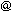hyde.uchicago.edu
 Webmaster: egaltsevakicp.uchicago.edu Last update: June 14, 2013